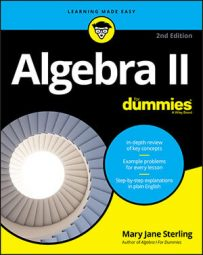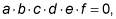##### Algebra II For DummiesThe multiplication property of zero is really useful for doing algebra. Of course, you may be thinking that multiplying by zero is no big deal. After all, zero times anything is zero, right? Well, that's exactly why it's a big deal. Read on...

You can use the multiplication property of zero when solving equations. If you can factor an equation — in other words, write it as the product of two or more multipliers — you can apply the multiplication property of zero to solve the equation.

The multiplication property of zero states that if the product ofat least one of the factors has to represent the number 0.

In other words, the only way the product of two or more values can be zero is for at least one of the values to actually be zero. If you multiply (16)(467)(11)(9)(0), the result is 0. It doesn't really matter what the other numbers are — the zero always wins.

The reason this property is so useful when solving equations is that if you want to solve the equation x7 – 16x5 + 5x4 – 80x2 = 0, for instance, you need the numbers that replace the x's to make the equation a true statement. This particular equation factors into x2(x3 + 5)(x – 4)(x + 4) = 0. The product of the four factors shown here is zero. The only way the product can be zero is if one or more of the factors is zero. For instance, if x = 4, the third factor is zero, and the whole product is zero. Also, if x is zero, the whole product is zero.